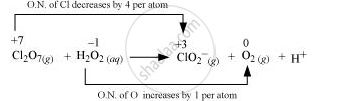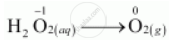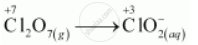CBSE (Science) Class 11CBSE
Share

# Balance the Following Equations in Basic Medium by Ion-electron Method and Oxidation Number Methods and Identify the Oxidising Agent and the Reducing Agent. - CBSE (Science) Class 11 - Chemistry

ConceptOxidation Number Balancing of Redox Reactions

#### Question

Balance the following equations in basic medium by ion-electron method and oxidation number methods and identify the oxidising agent and the reducing agent.

Cl_2O_(7(g)) + H_2O_(2(aq)) -> ClO_(2(aq))^- + O_(2(g)) + H_(aq)^+

#### SolutionThe oxidation number of Cl decreases from + 7 in Cl2O7 to + 3 in ClO_2^- and the oxidation number of O increases from – 1 in H2O2 to zero in O2. Hence, in this reaction, Cl2O7 is the oxidizing agent and H2O2 is the reducing agent.

Ion–electron method:

The oxidation half equation is:The oxidation number is balanced by adding 2 electrons as:

H_2O_(2(aq)) -> O_(2(g)) + 2e^(-)

The charge is balanced by adding 2OHions as:

H_2O_2(aq) -> + 2OH_((aq))^(-) -> O_(2(g)) + 2e^(-)

The oxygen atoms are balanced by adding 2H2O as:

H_2O_(2(aq)) + 2OH_(aq)^(-) -> O_(2(g)) + 2H_2O_(i) + 2e^(-)  ....(i)

The reduction half equation is:The Cl atoms are balanced as:

Cl_2O_(7(g)) + 8e^(-) -> 2ClO_(2(aq))^- + 6OH_((aq))^-

The oxygen atoms are balanced by adding 3H2O as:

Cl_2O_(7(g)) + 3H_2O_((l)) 8e^(-) ->  2ClO_(2(aq))^- + 6OH_(aq)^-   (ii)

The balanced equation can be obtained by multiplying equation (i) with 4 and adding equation (ii) to it as: Cl_2O_(7(g)) + 4H_2O_(2(aq)) + 2OH_(aq))^(-)  -> 2ClI_(2(aq))^(-) + 4O_(2(g)) + 5H_2O_(l)

Oxidation number method:

Total decrease in oxidation number of Cl2O= 4 × 2 = 8

Total increase in oxidation number of H2O2 = 2 × 1 = 2

By multiplying H2O2 and O2 with 4 to balance the increase and decrease in the oxidation number, we get:

Cl_2O_(7(g)) + 4H_2O_(2(aq)) -> ClO_(2(aq))^(-) + 4O_(2(g))

The Cl atoms are balanced as:

Cl_2O_(7(g)) + 4H_2O_(2(aq)) -> 2ClO_(2(aq))^-

+ 4O_(2(g))

The O atoms are balanced by adding 3H2O as:

Cl_2O_(7(g)) + 4H_2O_(2(aq)) -> 2ClO_(2(aq))^- + 4O_(2(g)) + 3H_2O_(l)

The H atoms are balanced by adding 2OH and 2H2O as:

Cl_2O_(7(g)) + 4H_2O_(2(aq)) + 2OH_((aq))^(-) -> 2ClO_(2(aq))^- + 4O_(2(g)) + 5H_2O_(l)

This is the required balanced equation.

Is there an error in this question or solution?

#### APPEARS IN

NCERT Solution for Chemistry Textbook for Class 11 (2018 to Current)
Chapter 8: Redox Reactions
Q: 19.3 | Page no. 274
Solution Balance the Following Equations in Basic Medium by Ion-electron Method and Oxidation Number Methods and Identify the Oxidising Agent and the Reducing Agent. Concept: Oxidation Number - Balancing of Redox Reactions.
S